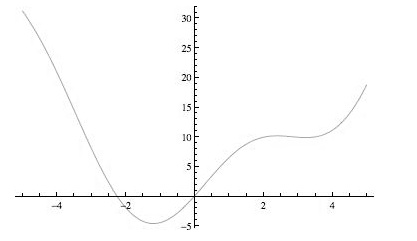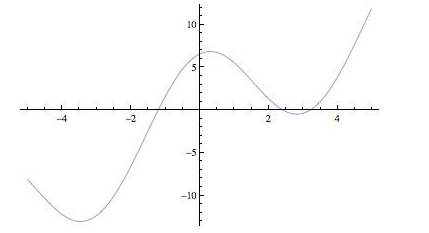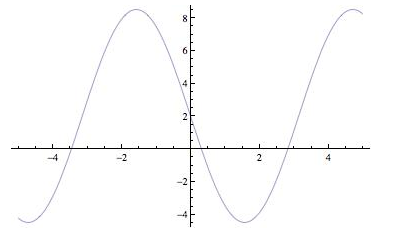# The intervals where the extreme value is increasing and decreasing.### Single Variable Calculus: Concepts...

4th Edition
James Stewart
Publisher: Cengage Learning
ISBN: 9781337687805### Single Variable Calculus: Concepts...

4th Edition
James Stewart
Publisher: Cengage Learning
ISBN: 9781337687805

#### Solutions

Chapter 4, Problem 18RE
To determine

## To find: The intervals where the extreme value is increasing and decreasing.

Expert Solution

### Explanation of Solution

Given information:

The function is f(x)=x2+6.5sinx .

Calculation:

Consider the equation.

f(x)=x2+6.5sinx

Differentiate above equation.

f(x)=2x+6.5cosx

And,

f(x)=26.5sinx

The graph f(x) is shown figure-1Figure-1

The absolute maximum value is f(1)=10

The absolute minimum value is f(1.8)=18

The graph f(x) is shown figure-2Figure-2

The graph f(x) is shown figure-3Figure-3

The function is decreasing for 5<x<1.25,2.5<x<3.25 and increasing on 1.25<x<2.5,3.25<x<5 and the inflection points for f(x) are (3.5,15),(0.3,2),(2.7,10)

### Have a homework question?

Subscribe to bartleby learn! Ask subject matter experts 30 homework questions each month. Plus, you’ll have access to millions of step-by-step textbook answers!